Home > CC2 > Chapter 7 > Lesson 7.1.7 > Problem7-82

7-82.
1. Ms. Poppy has finished grading her students’ tests. The scores were 62, 65, 93, 51, 55, 76, 79, 85, 55, 72, 78, 83, 91, and 82. Homework Help ✎

1. Find the median.

2. Find the quartiles.

3. Find the IQR.

4. Find the mean.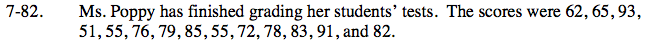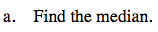Recall that the median is the middle number of an ordered set of data.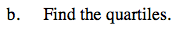Along with the median, the quartiles divide a set of data into four groups of the same size.

Lower Quartile (Q1) = 62
Upper Quartile (Q2) = 83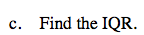IQR = Q3 – Q1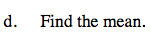Recall that the mean refers to the average of a set of data.